# Newton's laws of motionPage 1

#### WATCH ALL SLIDES

Slide 1## Newton's laws of motion

There are three of them.

They explain the motion of an object as resulting from the forces acting on the object.

Slide 2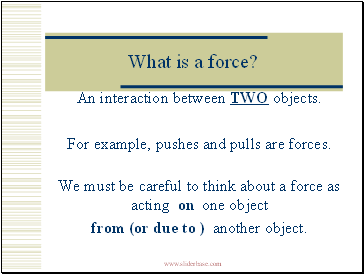What is a force?

An interaction between TWO objects.

For example, pushes and pulls are forces.

We must be careful to think about a force as acting on one object

from (or due to ) another object.

Slide 3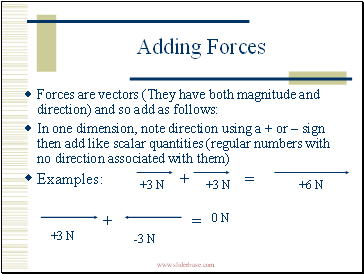Forces are vectors (They have both magnitude and direction) and so add as follows:

In one dimension, note direction using a + or – sign then add like scalar quantities (regular numbers with no direction associated with them)

Examples: + =

+ =

+3 N

+3 N

+6 N

+3 N

-3 N

0 N

Slide 4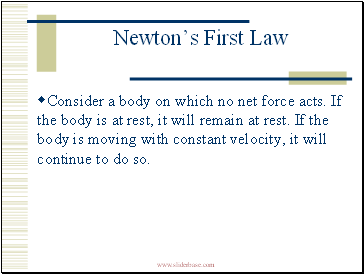## Newton’s First Law

Consider a body on which no net force acts. If the body is at rest, it will remain at rest. If the body is moving with constant velocity, it will continue to do so.

Slide 5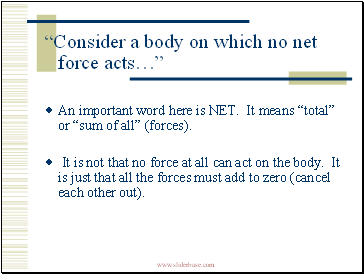“Consider a body on which no net force acts…”

An important word here is NET. It means “total” or “sum of all” (forces).

It is not that no force at all can act on the body. It is just that all the forces must add to zero (cancel each other out).

Slide 6Under this condition (no net force acting on the body):

If the body is at rest, it will remain at rest.

If the body is moving with constant velocity, it will continue to do so.

What if the body is moving with a velocity which is not constant? Why isn’t this discussed?

Slide 7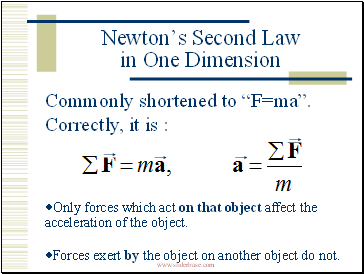## Newton’s Second Law in One Dimension

Commonly shortened to “F=ma”. Correctly, it is :

Only forces which act on that object affect the acceleration of the object.

Forces exert by the object on another object do not.

Slide 8Using Newton’s 2nd Law to Solve Problems

Identify all forces acting on the object

-Pushes or Pulls -Frictional forces -Tension in a string -Gravitational Force (or weight = mg where g is 9.8 m/s2) - “Normal forces” (one object touching another).

Draw a “Freebody Diagram” -draw the object, show all forces acting on that object as vectors pointing in the correct direction. Show the direction of the acceleration.

Chose a coordinate system.

Translate the freebody diagram into an algebraic expression based on Newton’s second law.

Go to page:
1  2